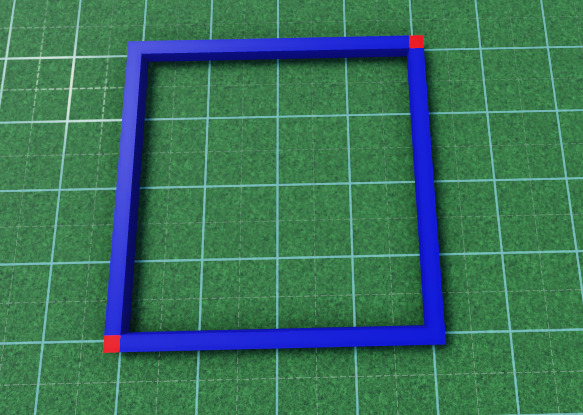# How to create 4 parts and size and position based on 2 points to create a box

I’m trying to create a build system, where players can place 2 points, and it’d create 4 walls and make it a square.

So, player places point A, and then places point B.

I need to figure out how to place parts like this.At the moment, I create the 4 parts, and then in a render stepped, as they move point B, it should size and position these parts as needed

``````for i = 1, 4 do
WallParts[i] = self:CreateWall(i)
end

-- inside a render loop
for _, wall in pairs(WallParts) do

end
``````

I know I could use like (point A.X - point B.X).Magnitude to get the straight line difference, but positioning them correctly is where I’m worried I’ll encounter issues

1 Like
``````local p1 = workspace.p1
local p2 = workspace.p2

local InbetweenVector = (workspace.p1.Position - workspace.p2.Position)
local IBVLength = InbetweenVector.Magnitude
local IBVDirection = InbetweenVector.Unit
local Center = p2.Position + ((IBVDirection * (IBVLength/2)))

local CenterVisualizePart = Instance.new("Part")
CenterVisualizePart.Anchored = true
CenterVisualizePart.Size = Vector3.new(1, 1, 1)
CenterVisualizePart.Position = Center
CenterVisualizePart.Parent = workspace

local LengthX = (p1.Position.X - Center.X)*2
local LXVisualizePart = Instance.new("Part")
LXVisualizePart.Anchored = true
LXVisualizePart.Size = Vector3.new(LengthX, 1, 1)
LXVisualizePart.Position = p1.Position - Vector3.new(LXVisualizePart.Size.X/2, 0, 0)
LXVisualizePart.Parent = workspace

local LengthZ = math.abs(p1.Position.Z - p2.Position.Z)
local LZVisualizePart = Instance.new("Part")
LZVisualizePart.Anchored = true
LZVisualizePart.Size = Vector3.new(1, 1, LengthZ)
LZVisualizePart.Position = LXVisualizePart.Position - Vector3.new(LXVisualizePart.Size.X/2, 0, 0) + Vector3.new(0, 0, LengthZ/2)
LZVisualizePart.Parent = workspace

local LX2VisualizePart = Instance.new("Part")
LX2VisualizePart.Anchored = true
LX2VisualizePart.Size = Vector3.new(LengthX, 1, 1)
LX2VisualizePart.Position = p1.Position - Vector3.new(LX2VisualizePart.Size.X/2, 0, 0) + Vector3.new(0, 0, LengthZ)
LX2VisualizePart.Parent = workspace

local LZ2VisualizePart = Instance.new("Part")
LZ2VisualizePart.Anchored = true
LZ2VisualizePart.Size = Vector3.new(1, 1, LengthZ)
LZ2VisualizePart.Position = LXVisualizePart.Position + Vector3.new(LXVisualizePart.Size.X/2, 0, 0) + Vector3.new(0, 0, LengthZ/2)
LZ2VisualizePart.Parent = workspace

p1.Position = p1.Position - (IBVDirection*7)
p2.Position = p2.Position + (IBVDirection*7)
``````

test.rbxl (33.4 KB)

this works but is very specific to the rotation & positions of the 2 blocks